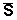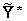# 交流电路供电单击或点击下面的示例电路以调用TINACloud并选择交互式DC模式以在线分析它们。

1. 瞬时功率： P（T） 是权力的时间函数， p（t）= u（t）* i（t）。 它是电压和电流的时间函数的乘积。 瞬时功率的定义适用于任何波形的信号。 的单位 瞬时功率 是VA。

2. 复数功率： S3. 真实成功 or 平均功率: P 可以用两种方式定义：作为复数幂的实部或作为 瞬时功率。 常规产品的 第二个定义比较笼统，因为我们可以用它来定义 瞬时功率 适用于任何信号波形，而不仅仅是正弦波。 在以下表达式中明确给出4. 无功功率： Q 是复杂力量的虚构部分。 单位为 伏安无功 （VAR）。 无功功率 积极归纳的 电路电容电路。 该功率仅限于正弦激励。 无功功率不做任何有用的工作或发热量， 是电路的电抗组件（电感器，电容器）返回电源的功率

5. 视在功率： S 是电压和电流均方根值的乘积，S = U * I。 视在功率的单位是VA。 的 视在功率 是绝对值 复杂的力量，因此仅定义为正弦激励。

###### 电源 因素 （COS φ)TINA的功率测量仪器还可以测量功率因数。= 3.9 ej38.7BмmA

PR我是2* R =（3.052+2.442）* 2 / 2 = 15.2 mW

QC = -I2/wC = -15.2 / 1.256 = -12.1mVARpV（t）= - vS（t）* i（t）= -10 cosωt* 3.9 cos（ωt + 38.7 м）= -39cosωt *（cosωt cos 38.7 м-sinωt sin 38.7 м ）= -30.45 cosωt + 24.4 sinωtVAS = V.S* I = 10 * 3.9 / 2 = 19.5 VA

 {TINA口译员的解决方案} OM：= 2 * PI * 1000; 五：= 10; I：= V /（R + 1 /（j * OM * C））; IAQ：= SQR（ABS（I））; PR：= IAQ * R / 2; PR = [15.3068m] QC：= IAQ /（OM * C * 2）; QC = [12.1808m] IC：=的Re（I）-j * IM（I）; SV：= - V * IC / 2; SV = [ - + 15.3068m * 12.1808m j]的

P QS R * I2 X *我2 ½Z½* I2 Z*I2 g ^ * V2 -B * V2 ½Y½* V2V2

#### 例如2

iS（t）=（100 * cosωt）mA w = 1 krad / s

Yeq= 1 / R + jωC + 1 / jωL = 1/5 + j250 * 10-6103 + 1 /（j * 20 * 10-3103）= 0.2 + j0.2 S.

½V ½= ½Z ½* I = I / ½Y ½= 0.1 / ê（0.2 + j0.2） ê= 0.3535 V.

P = V.2* G = 0.125 * 0.2 / 2 = 0.0125 W.

Q = -V2* B = – 0.125 * 0.2 / 2 = – 0.0125 varV =2*= 0.125 *（0.2-j0.2）/ 2 =（12.5 – j 12.5）mVA

S = V.2* Y = 0.125 * ê0.2 + j0.2 ê/ 2 = 0.01768 VA

cosφ= P / S = 0.707{TINA口译员的解决方案} OM：= 1000; 方法是：= 0.1; 五：= IS *（1 /（1 / R + J * OM * C + 1 /（j * OM * L）））; V = [250m-250m * j]的 S：= V *是/ 2; S = [12.5m-12.5m * j]的 警：=的Re（S）; 问：= IM（S）; P = [12.5m] Q = [ - 12.5m] ABS（S）= [17.6777m]{TINA口译员的解决方案！}
VS：= 100;
OM：= 1E8 * 2 * PI;
Ie:=Vs/(R2+1/j/om/C2+replus(replus(R1,j*om*L),1/j/om/C1));
Ze:=(R2+1/j/om/C2+replus(replus(R1,j*om*L),1/j/om/C1));

P = [14.6104]
Q = [ - 58.7055]

X欢迎来到 DesignSoft
如果需要任何帮助找到合适的产品或需要支持，可以进行聊天。